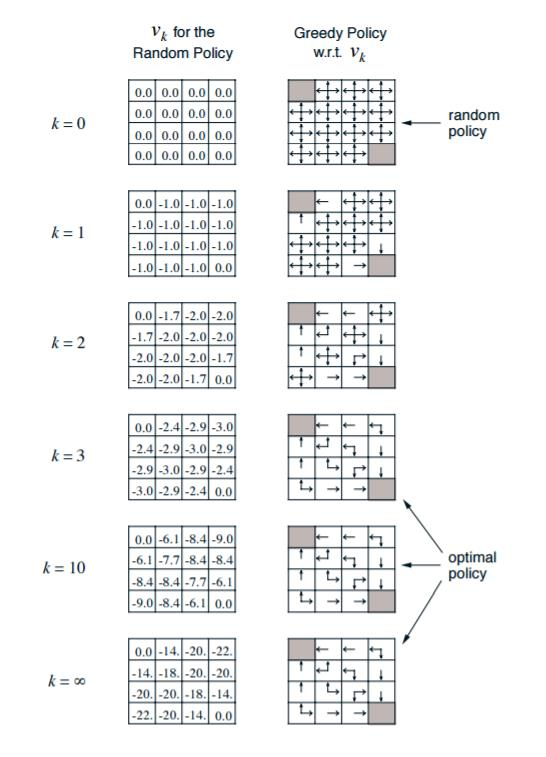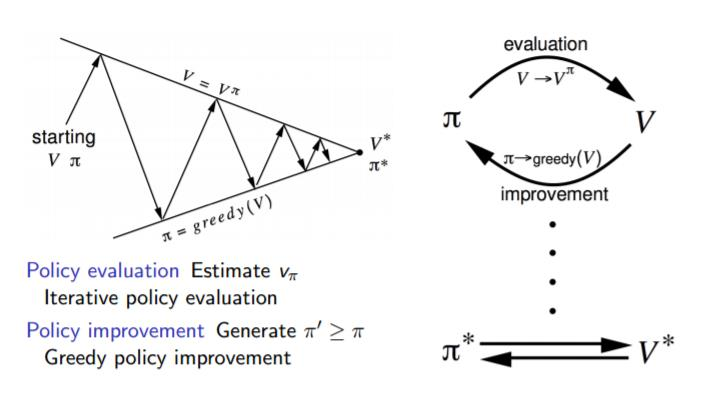# 强化学习（三）用动态规划（DP）求解

在强化学习（二）马尔科夫决策过程(MDP)中，我们讨论了用马尔科夫假设来简化强化学习模型的复杂度，这一篇我们在马尔科夫假设和贝尔曼方程的基础上讨论使用动态规划(Dynamic Programming, DP)来求解强化学习的问题。

动态规划这一篇对应Sutton书的第四章和UCL强化学习课程的第三讲。

# 1. 动态规划和强化学习问题的联系

对于动态规划，相信大家都很熟悉，很多使用算法的地方都会用到。就算是机器学习相关的算法，使用动态规划的也很多，比如之前讲到的隐马尔科夫模型HMM（二）前向后向算法评估观察序列概率隐马尔科夫模型HMM（四）维特比算法解码隐藏状态序列， 都是动态规划的典型例子。

动态规划的关键点有两个：一是问题的最优解可以由若干小问题的最优解构成，即通过寻找子问题的最优解来得到问题的最优解。第二是可以找到子问题状态之间的递推关系，通过较小的子问题状态递推出较大的子问题的状态。而强化学习的问题恰好是满足这两个条件的。

我们先看看强化学习的两个基本问题。

第一个问题是预测，即给定强化学习的6个要素：状态集$S$, 动作集$A$, 模型状态转化概率矩阵$P$, 即时奖励$R$，衰减因子$\gamma$,  给定策略$\pi$， 求解该策略的状态价值函数$v(\pi)$

第二个问题是控制，也就是求解最优的价值函数和策略。给定强化学习的5个要素：状态集$S$, 动作集$A$, 模型状态转化概率矩阵$P$, 即时奖励$R$，衰减因子$\gamma$, 求解最优的状态价值函数$v_{*}$和最优策略$\pi_{*}$

那么如何找到动态规划和强化学习这两个问题的关系呢？

回忆一下上一篇强化学习（二）马尔科夫决策过程(MDP)中状态价值函数的贝尔曼方程：$$v_{\pi}(s) = \sum\limits_{a \in A} \pi(a|s)(R_s^a + \gamma \sum\limits_{s' \in S}P_{ss'}^av_{\pi}(s'))$$

从这个式子我们可以看出，我们可以定义出子问题求解每个状态的状态价值函数，同时这个式子又是一个递推的式子, 意味着利用它，我们可以使用上一个迭代周期内的状态价值来计算更新当前迭代周期某状态$s$的状态价值。可见，使用动态规划来求解强化学习问题是比较自然的。

# 2. 策略评估求解预测问题

首先，我们来看如何使用动态规划来求解强化学习的预测问题，即求解给定策略的状态价值函数的问题。这个问题的求解过程我们通常叫做策略评估(Policy Evaluation)。

策略评估的基本思路是从任意一个状态价值函数开始，依据给定的策略，结合贝尔曼期望方程、状态转移概率和奖励同步迭代更新状态价值函数，直至其收敛，得到该策略下最终的状态价值函数。

假设我们在第$k$轮迭代已经计算出了所有的状态的状态价值，那么在第$k+1$轮我们可以利用第$k$轮计算出的状态价值计算出第$k+1$轮的状态价值。这是通过贝尔曼方程来完成的，即：$$v_{k+1}(s) = \sum\limits_{a \in A} \pi(a|s)(R_s^a + \gamma \sum\limits_{s' \in S}P_{ss'}^av_{k}(s'))$$

和上一节的式子唯一的区别是由于我们的策略$\pi$已经给定，我们不再写出，对应加上了迭代轮数的下标。我们每一轮可以对计算得到的新的状态价值函数再次进行迭代，直至状态价值的值改变很小(收敛)，那么我们就得出了预测问题的解，即给定策略的状态价值函数$v(\pi)$。

下面我们用一个具体的例子来说明策略评估的过程。

# 3. 策略评估求解实例

这是一个经典的Grid World的例子。我们有一个4x4的16宫格。只有左上和右下的格子是终止格子。该位置的价值固定为0，个体如果到达了该2个格子，则停止移动，此后每轮奖励都是0。个体在16宫格其他格的每次移动，得到的即时奖励$R$都是-1。注意个体每次只能移动一个格子，且只能上下左右4种移动选择，不能斜着走, 如果在边界格往外走，则会直接移动回到之前的边界格。衰减因子我们定义为$\gamma=1$。由于这里每次移动，下一格都是固定的，因此所有可行的的状态转化概率$P=1$。这里给定的策略是随机策略，即每个格子里有25%的概率向周围的4个格子移动。首先我们初始化所有格子的状态价值为0，如上图$k=0$的时候。现在我们开始策略迭代了。由于终止格子的价值固定为0，我们可以不将其加入迭代过程。在$k=1$的时候，我们利用上面的贝尔曼方程先计算第二行第一个格子的价值：$$v_1^{(21)} = \frac{1}{4}[(-1+0) +(-1+0)+(-1+0)+(-1+0)] = -1$$

第二行第二个格子的价值是：$$v_1^{(22)} = \frac{1}{4}[(-1+0) +(-1+0)+(-1+0)+(-1+0)] = -1$$

其他的格子都是类似的，第一轮的状态价值迭代的结果如上图$k=1$的时候。现在我们第一轮迭代完了。开始动态规划迭代第二轮了。还是看第二行第一个格子的价值：$$v_2^{(21)} = \frac{1}{4}[(-1+0) +(-1-1)+(-1-1)+(-1-1)] = -1.75$$

第二行第二个格子的价值是：$$v_2^{(22)} = \frac{1}{4}[(-1-1) +(-1-1)+(-1-1)+(-1-1)] = -2$$

最终得到的结果是上图$k=2$的时候。第三轮的迭代如下：

$$v_3^{(21)} = \frac{1}{4}[(-1-1.7) +(-1-2)+(-1-2)+(-1+0)] = -2.425$$$$v_3^{(22)} = \frac{1}{4}[(-1-1.7) +(-1-1.7)+(-1-2)+(-1-2)] = -2.85$$

最终得到的结果是上图$k=3$的时候。就这样一直迭代下去，直到每个格子的策略价值改变很小为止。这时我们就得到了所有格子的基于随机策略的状态价值。

可以看到，动态规划的策略评估计算过程并不复杂，但是如果我们的问题是一个非常复杂的模型的话，这个计算量还是非常大的。

# 4. 策略迭代求解控制问题

上面我们讲了使用策略评估求解预测问题，现在我们再来看如何使用动态规划求解强化学习的第二个问题控制问题。一种可行的方法就是根据我们之前基于任意一个给定策略评估得到的状态价值来及时调整我们的动作策略，这个方法我们叫做策略迭代(Policy Iteration)。

如何调整呢？最简单的方法就是贪婪法。考虑一种如下的贪婪策略：个体在某个状态下选择的行为是其能够到达后续所有可能的状态中状态价值最大的那个状态。还是以第三节的例子为例，如上面的图右边。当我们计算出最终的状态价值后，我们发现，第二行第一个格子周围的价值分别是0,-18,-20，此时我们用贪婪法，则我们调整行动策略为向状态价值为0的方向移动，而不是随机移动。也就是图中箭头向上。而此时第二行第二个格子周围的价值分别是-14,-14,-20, -20。那么我们整行动策略为向状态价值为-14的方向移动，也就是图中的向左向上。

如果用一副图来表示策略迭代的过程的话，如下图：在策略迭代过程中，我们循环进行两部分工作，第一步是使用当前策略$\pi_{*}$评估计算当前策略的最终状态价值$v_{*}$，第二步是根据状态价值$v_{*}$根据一定的方法（比如贪婪法）更新策略$\pi_{*}$，接着回到第一步，一直迭代下去，最终得到收敛的策略$\pi_{*}$和状态价值$v_{*}$。

# 5. 价值迭代求解控制问题

观察第三节的图发现，我们如果用贪婪法调整动作策略，那么当$k=3$的时候，我们就已经得到了最优的动作策略。而不用一直迭代到状态价值收敛才去调整策略。那么此时我们的策略迭代优化为价值迭代。

还是以第三节的例子为例，如上面的图右边。比如当$k=2$时，第二行第一个格子周围的价值分别是0,-2,-2，此时我们用贪婪法，则我们调整行动策略为向状态价值为0的方向移动，而不是随机移动。也就是图中箭头向上。而此时第二行第二个格子周围的价值分别是-1.7,-1.7,-2, -2。那么我们整行动策略为向状态价值为-1.7的方向移动，也就是图中的向左向上。

和上一节相比，我们没有等到状态价值收敛才调整策略，而是随着状态价值的迭代及时调整策略, 这样可以大大减少迭代次数。此时我们的状态价值的更新方法也和策略迭代不同。现在的贝尔曼方程迭代式子如下：$$v_{k+1}(s) = \max_{a \in A}(R_s^a + \gamma \sum\limits_{s' \in S}P_{ss'}^av_{k}(s'))$$

可见由于策略调整，我们现在价值每次更新倾向于贪婪法选择的最优策略对应的后续状态价值，这样收敛更快。

# 6. 异步动态规划算法

在前几节我们讲的都是同步动态规划算法，即每轮迭代我会计算出所有的状态价值并保存起来，在下一轮中，我们使用这些保存起来的状态价值来计算新一轮的状态价值。

另一种动态规划求解是异步动态规划算法，在这些算法里，每一次迭代并不对所有状态的价值进行更新，而是依据一定的原则有选择性的更新部分状态的价值，这类算法有自己的一些独特优势，当然有额会有一些额外的代价。

常见的异步动态规划算法有三种：

第一种是原位动态规划 (in-place dynamic programming)， 此时我们不会另外保存一份上一轮计算出的状态价值。而是即时计算即时更新。这样可以减少保存的状态价值的数量，节约内存。代价是收敛速度可能稍慢。

第二种是优先级动态规划 (prioritised sweeping)：该算法对每一个状态进行优先级分级，优先级越高的状态其状态价值优先得到更新。通常使用贝尔曼误差来评估状态的优先级，贝尔曼误差即新状态价值与前次计算得到的状态价值差的绝对值。这样可以加快收敛速度，代价是需要维护一个优先级队列。

第三种是实时动态规划 (real-time dynamic programming)：实时动态规划直接使用个体与环境交互产生的实际经历来更新状态价值，对于那些个体实际经历过的状态进行价值更新。这样个体经常访问过的状态将得到较高频次的价值更新，而与个体关系不密切、个体较少访问到的状态其价值得到更新的机会就较少。收敛速度可能稍慢。

# 7. 动态规划求解强化学习问题小结

动态规划是我们讲到的第一个系统求解强化学习预测和控制问题的方法。它的算法思路比较简单，主要就是利用贝尔曼方程来迭代更新状态价值，用贪婪法之类的方法迭代更新最优策略。

动态规划算法使用全宽度（full-width）的回溯机制来进行状态价值的更新，也就是说，无论是同步还是异步动态规划，在每一次回溯更新某一个状态的价值时，都要回溯到该状态的所有可能的后续状态，并利用贝尔曼方程更新该状态的价值。这种全宽度的价值更新方式对于状态数较少的强化学习问题还是比较有效的，但是当问题规模很大的时候，动态规划算法将会因贝尔曼维度灾难而无法使用。因此我们还需要寻找其他的针对复杂问题的强化学习问题求解方法。

下一篇我们讨论用蒙特卡罗方法来求解强化学习预测和控制问题的方法。

（欢迎转载，转载请注明出处。欢迎沟通交流： liujianping-ok@163.com）

posted @ 2018-08-12 20:36  刘建平Pinard  阅读(28320)  评论(80编辑  收藏